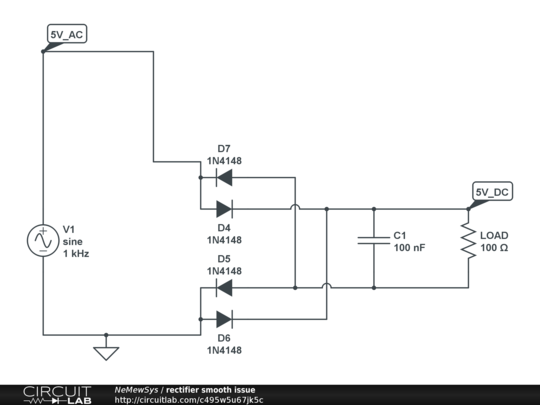# What seems to be the issue with this simple diode bridge rectifier with smooth capacitor?

I don't know if it is some limitation on the simulator, or my mistake, but I am trying to build this circuit:But this is what I get:For some reason, the voltage at the marker 5V_DC only gets one positive half-cycle, at a peak of 34.80mA. I was expecting 50mA 5v / 100ohm or 36mA [5v - 0.7v - 0.7v]/100ohm , not 34.80mA.

And the current at the LOAD is not smooth, despite the capacitor being there.

• You have grounded the wrong node in the circuit. Jul 11, 2017 at 16:11
• I made a similar misunderstanding on a breadboard before I understood how rectifiers really worked. All I knew then was AC-In, DC-Out. My application called for 170VDC, so I just rectified a 120VAC wall plug directly and then connected the negative side of that to a ground-referenced controller. As you can probably imagine, the circuit breaker (that I thought just had too much other stuff on it) and then the rectifier really didn't like that! A quick mental simulation showed the negative "DC" rail following the negative half-cycle all the way to -170V and back while the positive stayed at 0V. Jul 12, 2017 at 0:25

You have grounded the wrong point in your circuit.

If you monitor the bottom of your load resistor in the simulator you will get another surprise.simulate this circuit – Schematic created using CircuitLab

Figure 1. (a) In the positive half cycle D4 and D5 are in play. (b) In the negative half D6 and D7 are in play.

Ground at the bottom of C1 instead. Normally for a ground referenced DC supply like this we let the transformer "float" and ground the DC-.

And the current at the LOAD is not smooth, despite the capacitor being there.

You can get a good approximation of the discharge time by the RC time-constant: $\tau = RC = 100 \times 100n = 10 \; \mu s$. The capacitor will be discharged by 63% at this time. For 1 kHz (as in your schematic) you would need at least 100 times your C value. For 50/60 Hz you need 1,000 to 10,000 times that depending on your load.

• As a note, to fix this, I believe that you subtract the voltage at 5VDC-GND from the voltage on the other side of the load-GND Jul 11, 2017 at 18:16
• Sorry, I don't get your point. Can you elaborate? Jul 11, 2017 at 19:03

There are two significant problems with your circuit:

1. The capacitor is way too small. 100 nF with 100 Ω load has a time constant of only 10 µs.

2. You connected one side of the AC input to ground, and you then used that ground to measure the positive output against.

Your AC frequency is 1 kHz, so there are 500 µs between peaks. The labels on your graph are too small to read, so let's say the AC voltage is such that you get 15 V out of the rectifiers without load. If you want the drop to be no more than 1 V with 100 Ω load, then the capacitor needs to be (150 mA)(500 µs)/(1 V) = 75 µF. So 100 µF would be a good value for these made-up numbers. Note that this is 1000 times more than what you used.

Don't connect either side of the AC source to ground. Instead, connect the negative output of the rectifiers to ground. Then you can measure the positive output with respect to ground and get the output of the rectifiers.

Move your Gnd to V- as your 0V reference and ensure source is isolated(XFMR) otherwise it is offset from Vin 0V by Vf.

V and I then will be relative to the correct node then for load to Gnd.

## My rules of thumb

$$\text{For <10%pp load ripple use } T=RC=5 to 10 \times \text{input cycle time}$$ I prefer 8x for higher voltages where Vf is negligible.

Thus $$C>=8/(1\text{kHz}*100R)=80 \mu F$$

• for 5 Vp sine input 1kHz , R=100, C=80µF

• Vdc = 5-2*0.8V you can expect, around 0.7 to 1V drop per Si diode depending on power rating, where ESR above 0.6V (Si)

$$\text{ESR(diode)[Ω] = k/Pd[W] for constant k=0.5~1 typ. tolerance on diodes.}\tag4$$

You should intuitively expect 10% Vpp out ripple , causes at least 1/10% (=10x) input current ripple compared to Iout.

RC values affect both load ripple V and input ripple I, also both affect diode peak voltage drop.

$$V_{ dc(pk) }~~~= ~~V_{ dc(avg )} ~~~+~~ \frac{1}{2} V_{ o(pp)~}~~ \text{ ripple} ~~\tag5$$ or... $$(V_{ dc(pk) }~-~V_{ dc(avg)}~~~~)~~*2~~=~V_{ o(pp) }~~ \text{ ripple} ~~\tag6$$ $$\V_f=0.6V+ \dfrac{I_{ i(pk) }}{ k P_d}\tag6\$$

In order to charge quickly and discharge slowly with low ripple voltage ripple current is very high (inverse related). If Vf reaches 1V at peak current, consider higher power rating diodes with lower Vf.

Now you can try using 80uF.

• I get 5Vin(pk) - 2Vf + Vo(pk) and I assumed Vf=0.8V

• thus Vdc=3.4Vdc with 10% of Vdc = 340 mVpp sawtooth ripple

This guideline gets worse at lower voltages and low frequency as it implies huge caps.

Your expectation of exactly 0.7V drop per diode isn't realistic. 0.7V is just an often used approximation for standard silicon diodes, so having a little more or a little less isn't unexpected.

Your capacitor is only 100nF and your resistor is 100 Ohms. This gives a time constant of 10 microseconds, where as your 1khz waveform has a much longer half cycle period of 500 microseconds. The capacitor needs to be much larger for the smoothing you seek for the 1kHz frequency sine wave input. Try making it 100uF instead of 100nF.